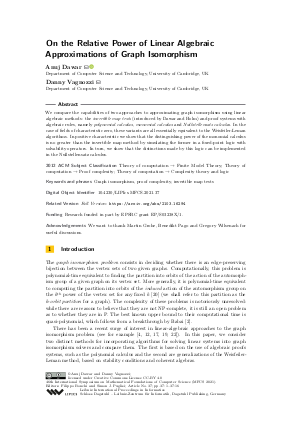Document# On the Relative Power of Linear Algebraic Approximations of Graph Isomorphism

### Authors Anuj Dawar, Danny Vagnozzi## File

LIPIcs.MFCS.2021.37.pdf
• Filesize: 0.8 MB
• 16 pages

## Acknowledgements

We want to thank Martin Grohe, Benedikt Pago and Gregory Wilsenach for useful discussions.

## Cite As

Anuj Dawar and Danny Vagnozzi. On the Relative Power of Linear Algebraic Approximations of Graph Isomorphism. In 46th International Symposium on Mathematical Foundations of Computer Science (MFCS 2021). Leibniz International Proceedings in Informatics (LIPIcs), Volume 202, pp. 37:1-37:16, Schloss Dagstuhl - Leibniz-Zentrum für Informatik (2021)
https://doi.org/10.4230/LIPIcs.MFCS.2021.37

## Abstract

We compare the capabilities of two approaches to approximating graph isomorphism using linear algebraic methods: the invertible map tests (introduced by Dawar and Holm) and proof systems with algebraic rules, namely polynomial calculus, monomial calculus and Nullstellensatz calculus. In the case of fields of characteristic zero, these variants are all essentially equivalent to the Weisfeiler-Leman algorithms. In positive characteristic we show that the distinguishing power of the monomial calculus is no greater than the invertible map method by simulating the former in a fixed-point logic with solvability operators. In turn, we show that the distinctions made by this logic can be implemented in the Nullstellensatz calculus.

## Subject Classification

##### ACM Subject Classification
• Theory of computation → Finite Model Theory
• Theory of computation → Proof complexity
• Theory of computation → Complexity theory and logic
##### Keywords
• Graph isomorphism
• proof complexity
• invertible map tests

## Metrics

• Access Statistics
• Total Accesses (updated on a weekly basis)
0

## References

1. A. Atserias, A. Bulatov, and A. Dawar. Affine systems of equations and counting infinitary logic. Theoretical Computer Science, 410(18):1666-1683, 2009. Automata, Languages and Programming (ICALP 2007).2. L. Babai. Graph isomorphism in quasipolynomial time [extended abstract]. In Proc. 48th Annual ACM SIGACT Symp. Theory of Computing, STOC, pages 684-697, 2016.3. P. Beame, R. Impagliazzo, J. Krajicek, T. Pitassi, and P. Pudlak. Lower bounds on Hilbert’s Nullstellensatz and propositional proofs. In Proceedings 35th Annual Symposium on Foundations of Computer Science, volume 73, pages 794-806, 1994.4. C. Berkholz and M. Grohe. Limitations of algebraic approaches to graph isomorphism testing. CoRR, abs/1502.05912, 2015.5. S. R. Buss. Lower bounds on Nullstellensatz proofs via designs. In in Proof Complexity and Feasible Arithmetics, P. Beame and S. Buss, eds., American Mathematical Society, pages 59-71. American Math. Soc, 1998.6. S. R. Buss, R. Impagliazzo, J. Krajícek, P. Pudlak, A. Razborov, and J. Sgall. Proof complexity in algebraic systems and bounded depth Frege systems with modular counting. Computational Complexity, 6:256-298, January 1997. URL: https://doi.org/10.1007/BF01294258.
7. J. Y. Cai, M. Fürer, and N. Immerman. An optimal lower bound on the number of variables for graph identification. Combinatorica, 12(4):389-410, 1992.8. M. Clegg, J. Edmonds, and R. Impagliazzo. Using the Gröbner basis algorithm to find proofs of unsatisfiability. Proceedings of STOC'96, March 2000.9. A. Dawar. The nature and power of fixed-point logic with counting. ACM SIGLOG News, 2:8-21, 2015.10. A. Dawar, E. Grädel, and W. Pakusa. Approximations of isomorphism and logics with linear algebraic operators. In 46th International Colloquium on Automata, Languages, and Programming, ICALP, pages 112:1-112:14, 2019. URL: https://doi.org/10.4230/LIPIcs.ICALP.2019.112.
11. A. Dawar, E. Graedel, B. Holm, E. Kopczyński, and W. Pakusa. Definability of linear equation systems over groups and rings. Logical Methods in Computer Science, 9, April 2012. URL: https://doi.org/10.2168/LMCS-9(4:12)2013.
12. A. Dawar and B. Holm. Pebble games with algebraic rules. In Artur Czumaj, Kurt Mehlhorn, Andrew Pitts, and Roger Wattenhofer, editors, Automata, Languages, and Programming, pages 251-262, Berlin, Heidelberg, 2012. Springer Berlin Heidelberg.13. A. Dawar and D. Vagnozzi. Generalizations of k-Weisfeiler-Leman stabilization. Moscow Journal of Number Theory and Combinatorics, 2020.14. A. Dawar and D. Vagnozzi. On the relative power of algebraic approximations of graph isomorphism. arXiv, 2021. URL: http://arxiv.org/abs/2103.16294.
15. C. de Seguins Pazzis. Invariance of simultaneous similarity and equivalence of matrices under extension of the ground field. Linear Algebra and its Applications, 433, February 2009. URL: https://doi.org/10.1016/j.laa.2010.03.022.
16. H-D. Ebbinghaus and J. Flum. Finite Model Theory. Springer, 2nd edition, 1999.17. E. Grädel, M. Grohe, B. Pago, and W. Pakusa. A finite-model-theoretic view on propositional proof complexity. Logical Methods in Computer Science, 15(1), 2019.18. B. Holm. Descriptive Complexity of Linear Algebra. PhD thesis, University of Cambridge, 2010.19. M. Lichter. Separating rank logic from polynomial time. In Proc. 36th ACM/IEEE Symp. on Logic in Computer Science (LICS), 2021.20. R. Mathon. A note on the graph isomorphism counting problem. Information Processing Letters, 8:131-136, 1979.21. M. Otto. Bounded Variable Logics and Counting - A Study in Finite Models, volume 9 of Lecture Notes in Logic. Springer-Verlag, 1997.22. G. Tinhofer. Graph isomorphism and theorems of Birkhoff type. Computing, 36:285-300, 1986.X

Feedback for Dagstuhl Publishing